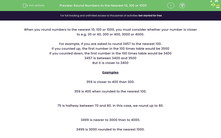# Round Numbers to the Nearest 10, 100 or 1,000

In this worksheet, students will revise rounding numbers to the nearest 10, 100 or 1,000.Key stage:  KS 3

Curriculum topic:   Number

Curriculum subtopic:   Round/Approximate Numbers Appropriately

Popular topics:   Rounding worksheets

Difficulty level:#### Worksheet Overview

When you round numbers to the nearest 10, 100 or 1,000, you need to consider whether your number is closer to e.g. 30 or 40, 300 or 400, 3,000 or 4,000.

For example, you might be asked to round 3,457 to the nearest 100.

If you counted up, the first number in the 100 times table would be 3,500

If you counted down, the first number in the 100 times table would be 3,400

3,457 is between 3,400 and 3,500

But it is closer to 3,500

The digit in the tens column is 5, so the number rounds up to 3,500 to the nearest 100.

Examples

359 is closer to 400 than 300.

The digit in the tens column is 5, so it rounds up to 400 to the nearest 100.

75 is halfway between 70 and 80.

In this case, we round up to 80 because the digit in the ones column is 5.

3,499 is nearer to 3,000 than to 4,000.

The digit in the hundreds column is 4, so we round down to 3,000 to the nearest 1,000.

It's all about the digit in the column to the right!

Let's have a go at the questions..### What is EdPlace?

We're your National Curriculum aligned online education content provider helping each child succeed in English, maths and science from year 1 to GCSE. With an EdPlace account you’ll be able to track and measure progress, helping each child achieve their best. We build confidence and attainment by personalising each child’s learning at a level that suits them.

Get started